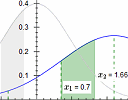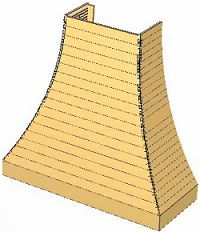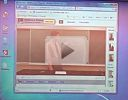Search IntMath
Close

450+ Math Lessons written by Math Professors and Teachers

5 Million+ Students Helped Each Year

1200+ Articles Written by Math Educators and Enthusiasts

Simplifying and Teaching Math for Over 23 Years

# IntMath Newsletter: z-Table interactive graph, CK-12 resource, range hood

By Murray Bourne, 04 Feb 2014

4 February 2014

1. New z-Table interactive graph
2. Resource: CK-12.org
3. Math puzzles
4. Friday math movie: Complex numbers in math class
5. Final thought: Giving

## 0. Sorry about the dummy mail

After I sent the last IntMath Newsletter, I made some updates to the script. When testing it, unfortunately some of my test mails (which contained text like "Lorem ipsum dolor sit amet") went out to a small number of you. I'm very sorry about that!

## 1. New z-Table interactive graphSome students get lost understanding what normal probability distributions are all about. You can investigate the meaning of the z-table using this new interactive graph. Normal Probability Distribution Graph Interactive

Here's some background on the topic:

Normal Probability Distribution

And here's some background on the new applet:

New z-Table interactive graph

## 2. Resource: CK-12.orgCK-12.org is a large collection of free math and science resources. There's lessons, self-testing quizzes, videos and interactive applets. As one of the students says: "It helps exceptionally with additional practice and study sessions outside of class time." — Kunal M.

It's worth a look!

## 3. Math puzzles

The puzzle in the last IntMath Newsletter asked about the percentage of pages in a book containing the number 5.

Correct answers with explanation were given by Michael, Nicos and Sai Krishna, and Sheldon had the right number of pages. It was interesting to see the different approaches taken by the solvers. Mathematics rarely has one "correct" way of going about things.

Also, some of you questioned whether "a 5" means "one 5 only" or "at least one 5". Interpreting math word questions is one of the biggest challenges people face - reading skills are important in math, too!

New math puzzle

I received a letter from John recently. His problem is a really interesting one because it involves many areas of mathematics. Here's what he wrote:

I am a consultant to cabinet manufacturers. I program their cabinet software to calculate and cutout their cabinet parts on CNC (computer numerical control) machines. A lot of cabinet companies create hoods that go over stoves that are curved on both sides and front. I need to calculate the joint of that curve as if it is laying flat. How do you calculate this?

Here is a picture of the hood.When viewed from the sides, the curves are arcs of circles, and the very top of the curve is vertical.

The width of the front face at the bottom is 60 cm, at the top is 30 cm wide and the (final) height of the curved portion is 50 cm.

Basically what I need is a formula to plot the Y coordinate of the curve given the X or vice-versa when the piece of sheet metal is laid out flat.

Once you have a solution, you are encouraged to make a model of it so you can be sure it works as expected. Take a photo of your model and upload it to Flickr, Dropbox or wherever, and embed the photo in your response (using something like <img src="LinkToYourImage">), or if that's too troublesome, email me your photo.

Leave your responses here.

## 4. Friday math movie: Complex numbers in math classThis is a very creative way to present a lesson - funny, too. Friday math movie: Complex numbers in math class

## 5. Final thought: Giving

Everyone is searching for happiness, and too many people try to find it in gadgets, cars, or other physical objects. Winston Churchill said:

We make a living by what we get, but we make a life by what we give. [Winston Churchill]

Until next time, enjoy whatever you learn.

See the 4 Comments below.

### 4 Comments on “IntMath Newsletter: z-Table interactive graph, CK-12 resource, range hood”

1. Pedington Peter Masi says:

I have problem about my calculation in linear algebra specific on the Gaussian & Gauss Jordan elimination method so i requested if could send me any maths software which could help me calculate such problems related to the topic.

2. Murray says:

@Pedington: This page may help: Inverse of a Matrix using Gauss-Jordan Elimination

You can't enter your own numbers, but it gives examples of finding inverses of 2x2, 3x3, 4x4 and 5x5 by Gauss-Jordan method.

3. Thomas Buckley says:

(Hi Murray,
This is my first attempt at html. Hoping that the equations
come out OK in your system. If not, will welcome comment).

Range Hood (Metric)

Range Hood (Metric)
We require PARTS of same radius cylinders joined
at 90°, so having a 45° mitre. Let p = length of arc of cylinders required. It can be shown that the flattened out shape of the joints will be a SINE WAVE. So, the solution for this edge or join shape should include a Sin, ( or Cosine) function.

For a hood of curved Height H, and curved Depth D, the radius of cylinders must be;

. . . Eq1

The length of cylinder arc required;.
( Note.This arc length is the same as the flattened length.)

. . . Eq2

Points for the curve can now be obtained, by calculating the value for y for x over the range of 0 to p.

. . Eq3

While Eq3 shows the Sine relationship and can be easily derived, this can be simplified to;

. . Eq4

For the given Range Hood of Height H=50cm, width at top 30cm and width at bottom 60cm. Curved Depth D =(60-30)/2 =15cm.

These values for H and D in Eq1 gives r = 90.833cm.
and putting D ,H and r in Eq2 gives p =52.948cm
Plotting the value of y, for a range of x having values 0 to 52.948 in Eq4 (or Eq3) will produce coordinates for the correct shape as per table.

 x   y     x    y     x      y
0   0 24  3.15 42    9.54
3   0.05  27   3.98  44     10.45
6   0.2   30   4.91  46     11.40
9   0.45  32   5.59  48     12.39
12  0.79  34   6.29  50     12.42
15  1.24  36   7.04  52     14.48
18  1.78  38   7.83  52.948 15.00
21  2.42  40   8.67

4. Murray says:

@Thomas: Thanks for your submission!

Just some notes about entering math equations and the table.

(1) For the equations, you used CodeCogs which is a good idea. Your response just had a link to the equations, and most readers will tend not to follow the links. In CodeCogs, below each equation you create, you'll see a yellow box that says "HTML code to embed this equation into a web page is:"

I copied the code in that yellow box for each of your equations.

For example, your first one was:

<a href="https://www.codecogs.com/eqnedit.php?latex=r=\frac{H^2+D^2}{2D}" target="_blank"><img src="https://latex.codecogs.com/gif.latex?r=\frac{H^2+D^2}{2D}" title="r=\frac{H^2+D^2}{2D}" /></a>

(2) In LaTeX, when you want "sin" or "arctan", by default they will appear as italics and so look like variables. You need to put a backslash in front so you get non-italic letters, like this: \sin and \arctan. I did so in the above examples.

(3) For a table, ordinary text won't line up nicely. One option is to create an actual HTML table, or (easier) put <pre> at the beginning and </pre> at the end of the table. The effect is "typewriter text", that is, monospaced.

(4) For the degrees sign, I use &deg;. What you put should have worked, but WordPress mangled it.

### Comment Preview

HTML: You can use simple tags like <b>, <a href="...">, etc.

To enter math, you can can either:

1. Use simple calculator-like input in the following format (surround your math in backticks, or qq on tablet or phone):
a^2 = sqrt(b^2 + c^2)
(See more on ASCIIMath syntax); or
2. Use simple LaTeX in the following format. Surround your math with $$ and $$.
$$\int g dx = \sqrt{\frac{a}{b}}$$
(This is standard simple LaTeX.)

NOTE: You can mix both types of math entry in your comment.